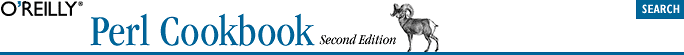home | O'Reilly's CD bookshelfs | FreeBSD | Linux | Cisco | Cisco Exam## 11.17. Program: Binary Trees

However, hashes provide no inherent ordering. To traverse the hash in a particular order, you must first extract its keys and then sort them. If you find yourself doing so many times, performance will suffer, but probably not enough to justify the time required to craft a fancy algorithm.

A tree structure provides ordered traversals. How do you write a tree in Perl? First, you grab one of your favorite textbooks on data structures; the authors recommend Cormen et al., as mentioned in Other Books in the Preface. Using an anonymous hash to represent each node in the tree, translate the algorithms in the book into Perl. This is usually much more straightforward than you would imagine.

The program code in Example 11-1 demonstrates an ordered binary tree implementation using anonymous hashes. Each node has three fields: a left child, a right child, and a value. The crucial property of an ordered binary tree is that at every node, all left children have values that are less than the current node value, and all right children have values that are greater.

The main program does three things. First, it creates a tree with 20 random nodes. Then it shows the in-order, pre-order, and post-order traversals of that tree. Finally, it allows the user to enter a key and reports whether that key is in the tree.

The insert function takes advantage of Perl's implicit pass-by-reference behavior on scalars to initialize an empty tree when asked to insert into an empty node. The assignment of the new node back to \$_ alters the value in its caller.

Although this data structure takes much more memory than a simple hash and the lookups are slower, the ordered traversals themselves are faster.

A B-Tree is not a binary tree; it is a more flexible tree structure normally maintained on disk. DB_File has a BTREE interface (see DB_File(3)), and Mark-Jason Dominus has an excellent article on B-Trees in The Perl Journal, Volume 2, Issue 4, Winter 1997, pp. 35-42.

If you want to learn more about binary trees, Introduction to Algorithms, by Cormen, Leiserson, and Rivest, and Algorithms in C, by Robert Sedgewick, both cover the basic material. But for a treatment of that material cast in native Perl, no book can compare with Mastering Algorithms with Perl, by Orwant, Hietaniemi, and MacDonald.

The program is shown in Example 11-1.

#### Example 11-1. bintree

```  #!/usr/bin/perl -w
# bintree - binary tree demo program
use strict;
my(\$root, \$n);
# first generate 20 random inserts
while (\$n++ < 20) { insert(\$root, int(rand(1000)))}
# now dump out the tree all three ways
print "Pre order:  ";  pre_order(\$root);  print "\n";
print "In order:   ";  in_order(\$root);   print "\n";
print "Post order: ";  post_order(\$root); print "\n";
# prompt until EOF
for (print "Search? "; <>; print "Search? ") {
chomp;
my \$found = search(\$root, \$_);
if (\$found) { print "Found \$_ at \$found, \$found->{VALUE}\n" }
else        { print "No \$_ in tree\n" }
}
exit;
#########################################
# insert given value into proper point of
# provided tree.  If no tree provided,
# use implicit pass by reference aspect of @_
# to fill one in for our caller.
sub insert {
my(\$tree, \$value) = @_;
unless (\$tree) {
\$tree = {  };                       # allocate new node
\$tree->{VALUE}  = \$value;
\$tree->{LEFT}   = undef;
\$tree->{RIGHT}  = undef;
\$_ = \$tree;                          # \$_ is reference param!
return;
}
if    (\$tree->{VALUE} > \$value) { insert(\$tree->{LEFT},  \$value) }
elsif (\$tree->{VALUE} < \$value) { insert(\$tree->{RIGHT}, \$value) }
else                            { warn "dup insert of \$value\n"  }
# XXX: no dups
}
# recurse on left child,
# then show current value,
# then recurse on right child.
sub in_order {
my(\$tree) = @_;
return unless \$tree;
in_order(\$tree->{LEFT});
print \$tree->{VALUE}, " ";
in_order(\$tree->{RIGHT});
}
# show current value,
# then recurse on left child,
# then recurse on right child.
sub pre_order {
my(\$tree) = @_;
return unless \$tree;
print \$tree->{VALUE}, " ";
pre_order(\$tree->{LEFT});
pre_order(\$tree->{RIGHT});
}
# recurse on left child,
# then recurse on right child,
# then show current value.
sub post_order {
my(\$tree) = @_;
return unless \$tree;
post_order(\$tree->{LEFT});
post_order(\$tree->{RIGHT});
print \$tree->{VALUE}, " ";
}
# find out whether provided value is in the tree.
# if so, return the node at which the value was found.
# cut down search time by only looking in the correct
# branch, based on current value.
sub search {
my(\$tree, \$value) = @_;
return unless \$tree;
if (\$tree->{VALUE} =  = \$value) {
return \$tree;
}
search(\$tree->{ (\$value < \$tree->{VALUE}) ? "LEFT" : "RIGHT"}, \$value)
}```